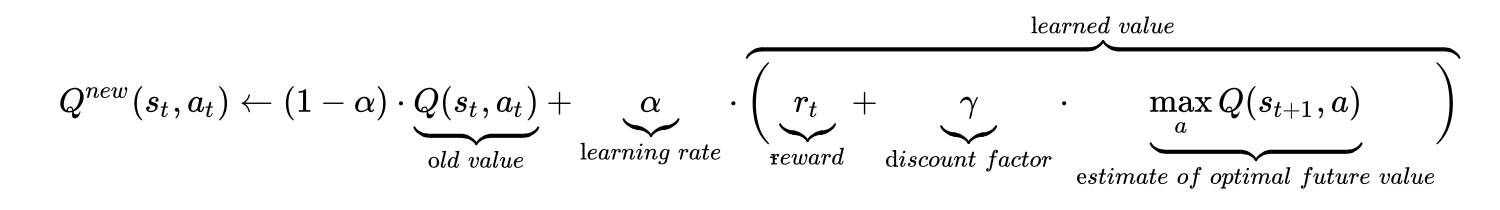# Q-learning

Q-learning is a model-free reinforcement learning technique. Specifically, Q-learning can be used to find an optimal action-selection policy for any given (finite) Markov decision process (MDP). It works by learning an action-value function that ultimately gives the expected utility of taking a given action in a given state and following the optimal policy thereafter. A policy is a rule that the agent follows in selecting actions, given the state it is in. When such an action-value function is learned, the optimal policy can be constructed by simply selecting the action with the highest value in each state. One of the strengths of Q-learning is that it is able to compare the expected utility of the available actions without requiring a model of the environment. Additionally, Q-learning can handle problems with stochastic transitions and rewards, without requiring any adaptations. It has been proven that for any finite MDP, Q-learning eventually finds an optimal policy, in the sense that the expected value of the total reward return over all successive steps, starting from the current state, is the maximum achievable.

Let Q be the quality of a picked action in function of the current state:
$latex Q:S\times A:\mapsto \mathbb{R}$
The learning process is described by:where the last term embodies the long-term reward. When the learning rate is zero the process is not future-driven. If it’s one, the process is only targetting the future rewards. Usually one take a value around 0.1. The discount factor gives an additional way to emphasize the future reward. Values around one or beyond give potentially diverging processes. Indeed, one can have all sorts of fluctuation like any other dynamical system.

Demonstrating q-learning with the gym environments is very easy. The well-known frozen-lake environment. The agent controls the movement of a character in a grid world. Some tiles of the grid are walkable, and others lead to the agent falling into the water. Additionally, the movement direction of the agent is uncertain and only partially depends on the chosen direction. The agent is rewarded for finding a walkable path to a goal tile.

import gym
import numpy as np
import random
import matplotlib.pyplot as plt
%matplotlib inline

#Initialize table with all zeros
Q = np.zeros([env.observation_space.n, env.action_space.n])
# Set learning parameters
lr = .8
y = .95
num_episodes = 2000
#create lists to contain total rewards and steps per episode
#jList = []
rList = []
for i in range(num_episodes):
#Reset environment and get first new observation
s = env.reset()
rAll = 0
d = False
j = 0
#The Q-Table learning algorithm
while j < 99:
j+=1
#Choose an action by greedily (with noise) picking from Q table
a = np.argmax(Q[s,:] + np.random.randn(1,env.action_space.n)*(1./(i+1)))
#Get new state and reward from environment
s1,r,d,_ = env.step(a)
#Update Q-Table with new knowledge
Q[s,a] = Q[s,a] + lr*(r + y*np.max(Q[s1,:]) - Q[s,a])
rAll += r
s = s1
if d == True:
break
#jList.append(j)
rList.append(rAll)

print("Score over time: " +  str(sum(rList)/num_episodes))
print("Final Q-Table Values")
print(Q)


Q-learning is computationally less complex than other approaches, one needs no accurate representation of the environment in order to be effective. This makes q-learning more fundamental than model-based methods. On the other hand, actual experiences need to be gathered in order for training, which makes exploration more dangerous. One cannot carry an explicit plan of how environmental dynamics affects the system, especially in response to an action previously taken.

Tags: